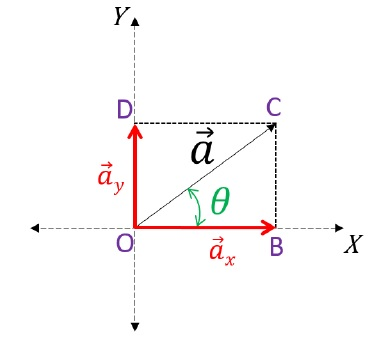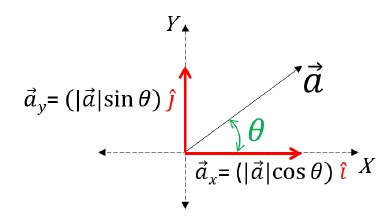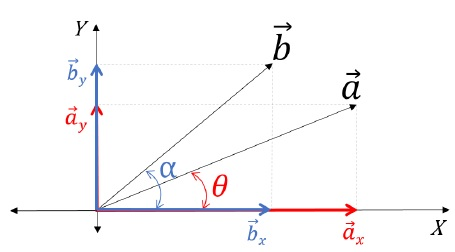> > Resolution of Vectors and Vector Addition

# Resolution of Vectors and Vector Addition

In contrast to the concept of addition, the concept of resolution of vectors could be well understood. For say, in the process of addition, let’s consider $$\vec{a}$$ and $$\vec{b}$$ are directly added to get $$\vec{S}$$. Now, let $$\vec{S}$$ be broken down to obtain $$\vec{a}$$ and $$\vec{b}$$ back. This is referred to as the process of resolution. Let us study the chapter resolution of vectors and vector addition in detail.

### Suggested  VideosScalar and vector quantitiesMotion in plane with constant accelerationUniform Circular Motion## Resolution of Vectors

A vector can be resolved into many different vectors, for resolution of vectors.  For Example: Let us consider two numbers, say, 4 and 6, which is further added to obtain 10. Further, now 10 is broken or resolved. However, the number 10 can also be resolved into many other numbers like  –10 = 5 + 5; 10 = 3 + 7 etc.

In a similar way, a vector can essentially be further broken or resolved to obtain multiple vectors of different magnitudes and directions. In Physics, vectors would be mainly resolved only along the coordinate axes, X, Y, and Z.

### Browse more Topics under Motion In A Plane### Resolving Vectors along X and Y Axis

To resolve a vector on an X-Y plane, first, draw the vector. Then, label and create the constructions on the figure as mentioned below :The complete figure seems to be like a parallelogram, further applying the Parallelogram law of vector addition. Herein, the two vectors $$\vec{a}_x$$ and $$\vec{a}_y$$ appears to be added by the parallelogram law of vector addition to obtain $$\vec{a}$$. Therefore, with this, we can say that $$\vec{a}_x$$ and $$\vec{a}_y$$ are the resolved output of $$\vec{a}$$ as $$\vec{a}$$ has been again broken back to its components. Here,

• $$\vec{a}_x$$ is the x-component; and
• $$\vec{a}_y$$ is the y-component of $$\vec{a}$$.

In order to find the magnitudes of each component, in △OBC:
cos θ = OBOC
Therefore, OB = OC cos θ
Hence, |$$\vec{a}_x$$ = |$$\vec{a}$$| cos θ [ Magnitude of x – component ]

sin θ = BCOC
So, BC = OC sin θ;
Also, BC = OD ( Opposite sides of Rectangle );
Therefore, |$$\vec{a}_y$$|  = |$$\vec{a}$$| sin θ [ Magnitude of y – component ]

Note

The General Rule of Thumb states that the subtended angle will always touch one of the components. Further, the component which the given angle touches or the given angle is subtended with will be the cos component of the given vector. Whereas the other will automatically be the sin component.

### Unit Vectors along Co-ordinate Axes

For the co-ordinate axes, there are special unit vectors designated by convention î, ĵ, and k̂, which respectively represents X, Y, and Z axes. By the property of unit vectors, |î| = |ĵ| = |k̂| = 1

### Writing Vectors in Component Form: Using Coordinate Axes Unit VectorsA vector can be expressed as a product of its magnitude and direction. Therefore,

• $$\vec{a}_x$$ = |$$\vec{a}$$| cos θ î. The magnitude of $$\vec{a}_x$$, which is |$$\vec{a}_x$$|, is multiplied to the direction in which $$\vec{a}_x$$ lies, which is the direction of x-axis, represented by the unit vector î.
• $$\vec{a}_y$$ = |$$\vec{a}$$| sin θ ĵ. The magnitude of $$\vec{a}_y$$, which is |$$\vec{a}_y$$|, is multiplied to the direction in which|$$\vec{a}_y$$| lies, which is the direction of y-axis, represented by the unit vector ĵ.
• Now we know that $$\vec{a}$$ = $$\vec{a}_x$$ + $$\vec{a}_y$$.

Therefore, $$\vec{a}$$ = (|$$\vec{a}$$| cos θ ) î + (|$$\vec{a}$$| sin θ ) ĵ.

### Addition of Vectors and Subtraction of Vectors in Component Form (Expressed as Unit Vectors)

Consider the following figure:Expressing $$\vec{a}$$ in component form,

• $$\vec{a}$$ = $$\vec{a}_x$$ + $$\vec{a}_y$$;
• $$\vec{a}$$ = |$$\vec{a}_x$$| î + |$$\vec{a}_y$$| ĵ;
• $$\vec{a}$$ = (|$$\vec{a}$$| cos θ ) î + (|$$\vec{a}$$| sin θ ) ĵ

Expressing $$\vec{b}$$ in component form,

• $$\vec{b}$$ = $$\vec{b}_x$$ + $$\vec{b}_y$$;
• $$\vec{b}$$ = |$$\vec{b}_x$$| î + |$$\vec{b}_y$$| ĵ;
• $$\vec{b}$$ = (|$$\vec{b}$$| cos α ) î + (|$$\vec{b}$$| sin α ) ĵ

Operation, (\vec{S}\) = $$\vec{a}$$ + $$\vec{b}$$;

• $$\vec{S}$$ = $$\vec{a}$$ + $$\vec{b}$$ = (|ax| î + |ay| ĵ) + (|bx| î + |by| ĵ);
• This implies, $$\vec{S}$$ = (|ax| + |bx|) î + (|ay| + |by|) ĵ;
• Hence, $$\vec{S}$$ = (|a| cos θ + |b| cos α) î + (|a| sin θ + |b| sin α) ĵ    [Final Sum]

Operation, $$\vec{S}$$ = $$\vec{a}$$ – $$\vec{b}$$;

• $$\vec{S}$$ = $$\vec{a}$$ – $$\vec{b}$$ = (|ax| î – |ay| ĵ) + (|bx| î – |by| ĵ);
• This implies, $$\vec{S}$$ = (|ax| – |bx|) î + (|ay| – |by|) ĵ;
• Hence, $$\vec{S}$$ = (|a| cos θ – |b| cos α) î + (|a| sin θ – |b| sin α) ĵ    [Final Difference]

## Solved Examples for You

Example: A laser beam is aimed 15.95° above the horizontal at a mirror 11,648 m away. It glances off the mirror and continues for an additional 8570. m at 11.44° above the horizon until it hits its target. What is the resultant displacement of the beam to the target?

Solution: Let us break up the vector into their components –

 x1 = r1 cos θ1 x1 = (11,648 m)cos(15.95°) x1 = 11,200 m y1 = r1 sin θ1 y1 = (11,648 m)sin(15.95°) y1 = 3,200 m
 x2 = r2 cos θ2 x2 = (8,570 m)cos(11.44°) x2 = 8,400 m y2 = r2 sin θ2 y2 = (8,570 m)sin(11.44°) y2 = 1,700 m

 x = 11,200 m + 8,400 m x = 19,600 m y = 3,200 m + 1,700 m y = 4,900 m

Add vectors at right angles with a combination of pythagorean theorem for magnitude…

 r = √(x2 + y2) r = √[(19,600 m)2 + (4,900 m)2] r = 20,200 m

and tangent for direction.

 tan θ = y = 4,900 m x 19,600 m θ = 14.04°
Share with friends

## Customize your course in 30 seconds

##### Which class are you in?
5th
6th
7th
8th
9th
10th
11th
12th
Get ready for all-new Live Classes!
Now learn Live with India's best teachers. Join courses with the best schedule and enjoy fun and interactive classes.Ashhar Firdausi
IIT Roorkee
BiologyDr. Nazma Shaik
VTU
ChemistryGaurav Tiwari
APJAKTU
Physics
Get Started

## Browse

##### Motion in a Plane1 Followers

Most reacted comment
2 Comment authorsRecent comment authors
Subscribe
Notify ofGuest
AWAIS

which situation we use vectors subtraction?Guest

When we give same magnitude but different direction then we use the substraction of method R bar=[p]-[Q] when its is whenever theta =180°Guest
optional

What are there applications in real life?

## Question Mark?

Have a doubt at 3 am? Our experts are available 24x7. Connect with a tutor instantly and get your concepts cleared in less than 3 steps.